## 5.315. path

Origin
Constraint

$\mathrm{𝚙𝚊𝚝𝚑}\left(\mathrm{𝙽𝙿𝙰𝚃𝙷},\mathrm{𝙽𝙾𝙳𝙴𝚂}\right)$

Arguments
 $\mathrm{𝙽𝙿𝙰𝚃𝙷}$ $\mathrm{𝚍𝚟𝚊𝚛}$ $\mathrm{𝙽𝙾𝙳𝙴𝚂}$ $\mathrm{𝚌𝚘𝚕𝚕𝚎𝚌𝚝𝚒𝚘𝚗}\left(\mathrm{𝚒𝚗𝚍𝚎𝚡}-\mathrm{𝚒𝚗𝚝},\mathrm{𝚜𝚞𝚌𝚌}-\mathrm{𝚍𝚟𝚊𝚛}\right)$
Restrictions
 $\mathrm{𝙽𝙿𝙰𝚃𝙷}\ge 1$ $\mathrm{𝙽𝙿𝙰𝚃𝙷}\le |\mathrm{𝙽𝙾𝙳𝙴𝚂}|$ $\mathrm{𝚛𝚎𝚚𝚞𝚒𝚛𝚎𝚍}$$\left(\mathrm{𝙽𝙾𝙳𝙴𝚂},\left[\mathrm{𝚒𝚗𝚍𝚎𝚡},\mathrm{𝚜𝚞𝚌𝚌}\right]\right)$ $|\mathrm{𝙽𝙾𝙳𝙴𝚂}|>0$ $\mathrm{𝙽𝙾𝙳𝙴𝚂}.\mathrm{𝚒𝚗𝚍𝚎𝚡}\ge 1$ $\mathrm{𝙽𝙾𝙳𝙴𝚂}.\mathrm{𝚒𝚗𝚍𝚎𝚡}\le |\mathrm{𝙽𝙾𝙳𝙴𝚂}|$ $\mathrm{𝚍𝚒𝚜𝚝𝚒𝚗𝚌𝚝}$$\left(\mathrm{𝙽𝙾𝙳𝙴𝚂},\mathrm{𝚒𝚗𝚍𝚎𝚡}\right)$ $\mathrm{𝙽𝙾𝙳𝙴𝚂}.\mathrm{𝚜𝚞𝚌𝚌}\ge 1$ $\mathrm{𝙽𝙾𝙳𝙴𝚂}.\mathrm{𝚜𝚞𝚌𝚌}\le |\mathrm{𝙽𝙾𝙳𝙴𝚂}|$
Purpose

Cover the digraph $G$ described by the $\mathrm{𝙽𝙾𝙳𝙴𝚂}$ collection with $\mathrm{𝙽𝙿𝙰𝚃𝙷}$ paths in such a way that each vertex of $G$ belongs to exactly one path.

Example
 $\left(\begin{array}{c}3,〈\begin{array}{cc}\mathrm{𝚒𝚗𝚍𝚎𝚡}-1\hfill & \mathrm{𝚜𝚞𝚌𝚌}-1,\hfill \\ \mathrm{𝚒𝚗𝚍𝚎𝚡}-2\hfill & \mathrm{𝚜𝚞𝚌𝚌}-3,\hfill \\ \mathrm{𝚒𝚗𝚍𝚎𝚡}-3\hfill & \mathrm{𝚜𝚞𝚌𝚌}-5,\hfill \\ \mathrm{𝚒𝚗𝚍𝚎𝚡}-4\hfill & \mathrm{𝚜𝚞𝚌𝚌}-7,\hfill \\ \mathrm{𝚒𝚗𝚍𝚎𝚡}-5\hfill & \mathrm{𝚜𝚞𝚌𝚌}-1,\hfill \\ \mathrm{𝚒𝚗𝚍𝚎𝚡}-6\hfill & \mathrm{𝚜𝚞𝚌𝚌}-6,\hfill \\ \mathrm{𝚒𝚗𝚍𝚎𝚡}-7\hfill & \mathrm{𝚜𝚞𝚌𝚌}-7,\hfill \\ \mathrm{𝚒𝚗𝚍𝚎𝚡}-8\hfill & \mathrm{𝚜𝚞𝚌𝚌}-6\hfill \end{array}〉\hfill \end{array}\right)$ $\left(\begin{array}{c}1,〈\begin{array}{cc}\mathrm{𝚒𝚗𝚍𝚎𝚡}-1\hfill & \mathrm{𝚜𝚞𝚌𝚌}-8,\hfill \\ \mathrm{𝚒𝚗𝚍𝚎𝚡}-2\hfill & \mathrm{𝚜𝚞𝚌𝚌}-7,\hfill \\ \mathrm{𝚒𝚗𝚍𝚎𝚡}-3\hfill & \mathrm{𝚜𝚞𝚌𝚌}-6,\hfill \\ \mathrm{𝚒𝚗𝚍𝚎𝚡}-4\hfill & \mathrm{𝚜𝚞𝚌𝚌}-5,\hfill \\ \mathrm{𝚒𝚗𝚍𝚎𝚡}-5\hfill & \mathrm{𝚜𝚞𝚌𝚌}-5,\hfill \\ \mathrm{𝚒𝚗𝚍𝚎𝚡}-6\hfill & \mathrm{𝚜𝚞𝚌𝚌}-4,\hfill \\ \mathrm{𝚒𝚗𝚍𝚎𝚡}-7\hfill & \mathrm{𝚜𝚞𝚌𝚌}-3,\hfill \\ \mathrm{𝚒𝚗𝚍𝚎𝚡}-8\hfill & \mathrm{𝚜𝚞𝚌𝚌}-2\hfill \end{array}〉\hfill \end{array}\right)$ $\left(\begin{array}{c}8,〈\begin{array}{cc}\mathrm{𝚒𝚗𝚍𝚎𝚡}-1\hfill & \mathrm{𝚜𝚞𝚌𝚌}-1,\hfill \\ \mathrm{𝚒𝚗𝚍𝚎𝚡}-2\hfill & \mathrm{𝚜𝚞𝚌𝚌}-2,\hfill \\ \mathrm{𝚒𝚗𝚍𝚎𝚡}-3\hfill & \mathrm{𝚜𝚞𝚌𝚌}-3,\hfill \\ \mathrm{𝚒𝚗𝚍𝚎𝚡}-4\hfill & \mathrm{𝚜𝚞𝚌𝚌}-4,\hfill \\ \mathrm{𝚒𝚗𝚍𝚎𝚡}-5\hfill & \mathrm{𝚜𝚞𝚌𝚌}-5,\hfill \\ \mathrm{𝚒𝚗𝚍𝚎𝚡}-6\hfill & \mathrm{𝚜𝚞𝚌𝚌}-6,\hfill \\ \mathrm{𝚒𝚗𝚍𝚎𝚡}-7\hfill & \mathrm{𝚜𝚞𝚌𝚌}-7,\hfill \\ \mathrm{𝚒𝚗𝚍𝚎𝚡}-8\hfill & \mathrm{𝚜𝚞𝚌𝚌}-8\hfill \end{array}〉\hfill \end{array}\right)$

The first $\mathrm{𝚙𝚊𝚝𝚑}$ constraint holds since its second argument corresponds to the 3 (i.e., the first argument of the $\mathrm{𝚙𝚊𝚝𝚑}$ constraint) paths depicted by Figure 5.315.1.

##### Figure 5.315.1. The three paths corresponding to the first example of the Example slot; each vertex contains the information $\mathrm{𝚒𝚗𝚍𝚎𝚡}|\mathrm{𝚜𝚞𝚌𝚌}$ where $\mathrm{𝚜𝚞𝚌𝚌}$ is the index of its successor in the path (by convention one of the extremities of a path points to itself).Typical
 $\mathrm{𝙽𝙿𝙰𝚃𝙷}<|\mathrm{𝙽𝙾𝙳𝙴𝚂}|$ $|\mathrm{𝙽𝙾𝙳𝙴𝚂}|>1$
Symmetry

Items of $\mathrm{𝙽𝙾𝙳𝙴𝚂}$ are permutable.

Arg. properties

Functional dependency: $\mathrm{𝙽𝙿𝙰𝚃𝙷}$ determined by $\mathrm{𝙽𝙾𝙳𝙴𝚂}$.

Reformulation

The $\mathrm{𝚙𝚊𝚝𝚑}$ constraint can be expressed in term of (1) a set of ${|\mathrm{𝙽𝙾𝙳𝙴𝚂}|}^{2}$ reified constraints for avoiding circuit between more than one node and of (2) $|\mathrm{𝙽𝙾𝙳𝙴𝚂}|$ reified constraints and of one sum constraint for counting the paths and of (3) a set of ${|\mathrm{𝙽𝙾𝙳𝙴𝚂}|}^{2}$ reified constraints and of $|\mathrm{𝙽𝙾𝙳𝙴𝚂}|$ inequalities constraints for enforcing the fact that each vertex has at most two children.

1. For each vertex $\mathrm{𝙽𝙾𝙳𝙴𝚂}\left[i\right]$ $\left(i\in \left[1,|\mathrm{𝙽𝙾𝙳𝙴𝚂}|\right]\right)$ of the $\mathrm{𝙽𝙾𝙳𝙴𝚂}$ collection we create a variable ${R}_{i}$ that takes its value within interval $\left[1,|\mathrm{𝙽𝙾𝙳𝙴𝚂}|\right]$. This variable represents the rank of vertex $\mathrm{𝙽𝙾𝙳𝙴𝚂}\left[i\right]$ within a solution. It is used to prevent the creation of circuit involving more than one vertex as explained now. For each pair of vertices $\mathrm{𝙽𝙾𝙳𝙴𝚂}\left[i\right],\mathrm{𝙽𝙾𝙳𝙴𝚂}\left[j\right]$ $\left(i,j\in \left[1,|\mathrm{𝙽𝙾𝙳𝙴𝚂}|\right]\right)$ of the $\mathrm{𝙽𝙾𝙳𝙴𝚂}$ collection we create a reified constraint of the form $\mathrm{𝙽𝙾𝙳𝙴𝚂}\left[i\right].\mathrm{𝚜𝚞𝚌𝚌}=\mathrm{𝙽𝙾𝙳𝙴𝚂}\left[j\right].\mathrm{𝚒𝚗𝚍𝚎𝚡}\wedge i\ne j⇒{R}_{i}<{R}_{j}$. The purpose of this constraint is to express the fact that, if there is an arc from vertex $\mathrm{𝙽𝙾𝙳𝙴𝚂}\left[i\right]$ to another vertex $\mathrm{𝙽𝙾𝙳𝙴𝚂}\left[j\right]$, then ${R}_{i}$ should be strictly less than ${R}_{j}$.

2. For each vertex $\mathrm{𝙽𝙾𝙳𝙴𝚂}\left[i\right]$ $\left(i\in \left[1,|\mathrm{𝙽𝙾𝙳𝙴𝚂}|\right]\right)$ of the $\mathrm{𝙽𝙾𝙳𝙴𝚂}$ collection we create a 0-1 variable ${B}_{i}$ and state the following reified constraint $\mathrm{𝙽𝙾𝙳𝙴𝚂}\left[i\right].\mathrm{𝚜𝚞𝚌𝚌}=\mathrm{𝙽𝙾𝙳𝙴𝚂}\left[i\right].\mathrm{𝚒𝚗𝚍𝚎𝚡}⇔{B}_{i}$ in order to force variable ${B}_{i}$ to be set to value 1 if and only if there is a loop on vertex $\mathrm{𝙽𝙾𝙳𝙴𝚂}\left[i\right]$. Finally we create a constraint $\mathrm{𝙽𝙿𝙰𝚃𝙷}={B}_{1}+{B}_{2}+\cdots +{B}_{|\mathrm{𝙽𝙾𝙳𝙴𝚂}|}$ for stating the fact that the number of paths is equal to the number of loops of the graph.

3. For each pair of vertices $\mathrm{𝙽𝙾𝙳𝙴𝚂}\left[i\right],\mathrm{𝙽𝙾𝙳𝙴𝚂}\left[j\right]$ $\left(i,j\in \left[1,|\mathrm{𝙽𝙾𝙳𝙴𝚂}|\right]\right)$ of the $\mathrm{𝙽𝙾𝙳𝙴𝚂}$ collection we create a 0-1 variable ${B}_{ij}$ and state the following reified constraint $\mathrm{𝙽𝙾𝙳𝙴𝚂}\left[i\right].\mathrm{𝚜𝚞𝚌𝚌}=\mathrm{𝙽𝙾𝙳𝙴𝚂}\left[j\right].\mathrm{𝚒𝚗𝚍𝚎𝚡}\wedge i\ne j⇔{B}_{ij}$. Variable ${B}_{ij}$ is set to value 1 if and only if there is an arc from $\mathrm{𝙽𝙾𝙳𝙴𝚂}\left[i\right]$ to $\mathrm{𝙽𝙾𝙳𝙴𝚂}\left[j\right]$. Then for each vertex $\mathrm{𝙽𝙾𝙳𝙴𝚂}\left[j\right]$ $\left(j\in \left[1,|\mathrm{𝙽𝙾𝙳𝙴𝚂}|\right]\right)$ we create a constraint of the form ${B}_{1j}+{B}_{2j}+\cdots +{B}_{|\mathrm{𝙽𝙾𝙳𝙴𝚂}|j}\le 1$.

Counting
 Length ($n$) 2 3 4 5 6 7 8 Solutions 3 13 73 501 4051 37633 394353

Number of solutions for $\mathrm{𝚙𝚊𝚝𝚑}$: domains $0..n$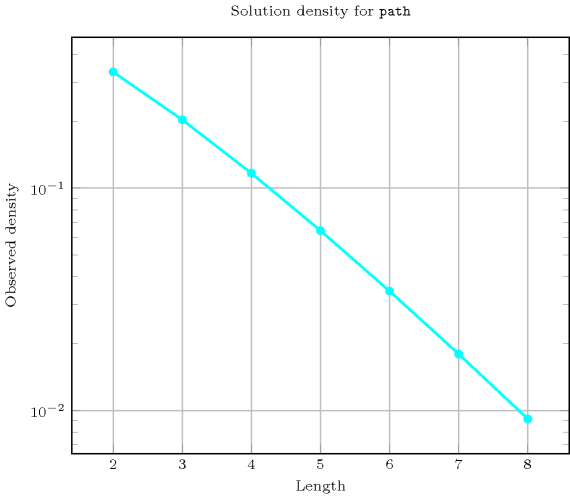Length ($n$)2345678
Total31373501405137633394353
 Parameter value

12624120720504040320
21636240180015120141120
3-112120120012600141120
4--120300420058800
5---13063011760
6----1421176
7-----156
8------1

Solution count for $\mathrm{𝚙𝚊𝚝𝚑}$: domains $0..n$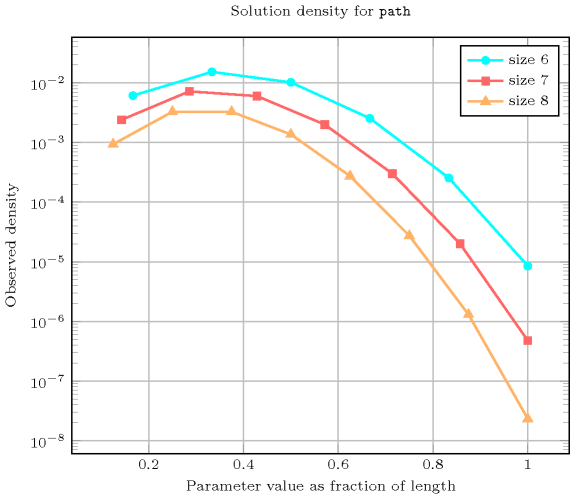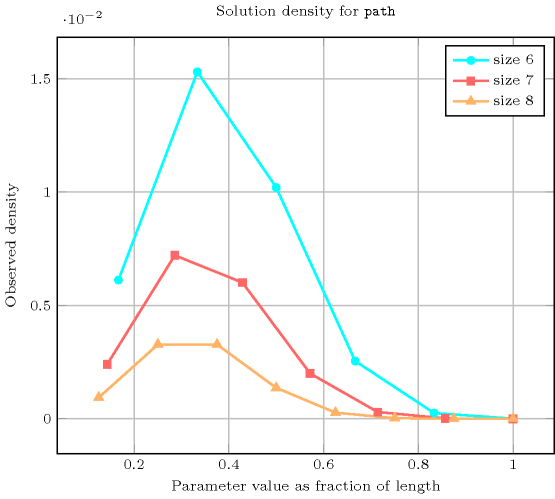common keyword: $\mathrm{𝚌𝚒𝚛𝚌𝚞𝚒𝚝}$ (graph partitioning constraint, one_succ), $\mathrm{𝚍𝚘𝚖}_\mathrm{𝚛𝚎𝚊𝚌𝚑𝚊𝚋𝚒𝚕𝚒𝚝𝚢}$ (path), $\mathrm{𝚙𝚊𝚝𝚑}_\mathrm{𝚏𝚛𝚘𝚖}_\mathrm{𝚝𝚘}$ (path, select an induced subgraph so that there is a path from a given vertex to an other given vertex), $\mathrm{𝚙𝚛𝚘𝚙𝚎𝚛}_\mathrm{𝚌𝚒𝚛𝚌𝚞𝚒𝚝}$ (graph partitioning constraint, one_succ).

generalisation: $\mathrm{𝚋𝚒𝚗𝚊𝚛𝚢}_\mathrm{𝚝𝚛𝚎𝚎}$ (at most one child replaced by at most two children), $\mathrm{𝚝𝚎𝚖𝚙𝚘𝚛𝚊𝚕}_\mathrm{𝚙𝚊𝚝𝚑}$ (vertices are located in time, and to each arc corresponds a precedence constraint), $\mathrm{𝚝𝚛𝚎𝚎}$ (at most one child replaced by no limit on the number of children).

related: $\mathrm{𝚋𝚊𝚕𝚊𝚗𝚌𝚎}_\mathrm{𝚙𝚊𝚝𝚑}$ (counting number of paths versus controlling how balanced the paths are).

Keywords
Arc input(s)

$\mathrm{𝙽𝙾𝙳𝙴𝚂}$

Arc generator
$\mathrm{𝐶𝐿𝐼𝑄𝑈𝐸}$$↦\mathrm{𝚌𝚘𝚕𝚕𝚎𝚌𝚝𝚒𝚘𝚗}\left(\mathrm{𝚗𝚘𝚍𝚎𝚜}\mathtt{1},\mathrm{𝚗𝚘𝚍𝚎𝚜}\mathtt{2}\right)$

Arc arity
Arc constraint(s)
$\mathrm{𝚗𝚘𝚍𝚎𝚜}\mathtt{1}.\mathrm{𝚜𝚞𝚌𝚌}=\mathrm{𝚗𝚘𝚍𝚎𝚜}\mathtt{2}.\mathrm{𝚒𝚗𝚍𝚎𝚡}$
Graph property(ies)
 $•$$\mathrm{𝐌𝐀𝐗}_\mathrm{𝐍𝐒𝐂𝐂}$$\le 1$ $•$$\mathrm{𝐍𝐂𝐂}$$=\mathrm{𝙽𝙿𝙰𝚃𝙷}$ $•$$\mathrm{𝐌𝐀𝐗}_\mathrm{𝐈𝐃}$$\le 1$

Graph class
$\mathrm{𝙾𝙽𝙴}_\mathrm{𝚂𝚄𝙲𝙲}$

Graph model

We use the same graph constraint as for the $\mathrm{𝚋𝚒𝚗𝚊𝚛𝚢}_\mathrm{𝚝𝚛𝚎𝚎}$ constraint, except that we replace the graph property $\mathrm{𝐌𝐀𝐗}_\mathrm{𝐈𝐃}$$\le 2$, which constraints the maximum in-degree of the final graph to not exceed 2 by $\mathrm{𝐌𝐀𝐗}_\mathrm{𝐈𝐃}$$\le 1$. $\mathrm{𝐌𝐀𝐗}_\mathrm{𝐈𝐃}$ does not consider loops: This is why we do not have any problem with the final node of each path.

Parts (A) and (B) of Figure 5.315.2 respectively show the initial and final graph associated with the first example of the Example slot. Since we use the $\mathrm{𝐍𝐂𝐂}$ graph property, we display the three connected components of the final graph. Each of them corresponds to a path. Since we use the $\mathrm{𝐌𝐀𝐗}_\mathrm{𝐈𝐃}$ graph property, we also show with a double circle a vertex that has a maximum number of predecessors.

The $\mathrm{𝚙𝚊𝚝𝚑}$ constraint holds since all strongly connected components of the final graph have no more than one vertex, since $\mathrm{𝙽𝙿𝙰𝚃𝙷}=$$\mathrm{𝐍𝐂𝐂}$$=3$ and since $\mathrm{𝐌𝐀𝐗}_\mathrm{𝐈𝐃}$$=1$.

##### Figure 5.315.2. Initial and final graph of the $\mathrm{𝚙𝚊𝚝𝚑}$ constraint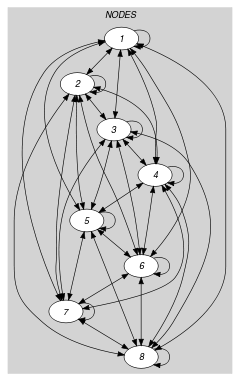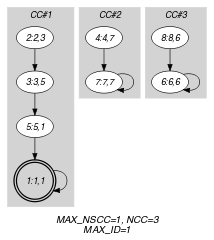(a) (b)